Question

# When a 15.6 mL sample of a 0.498 M aqueous acetic acid solution is titrated with...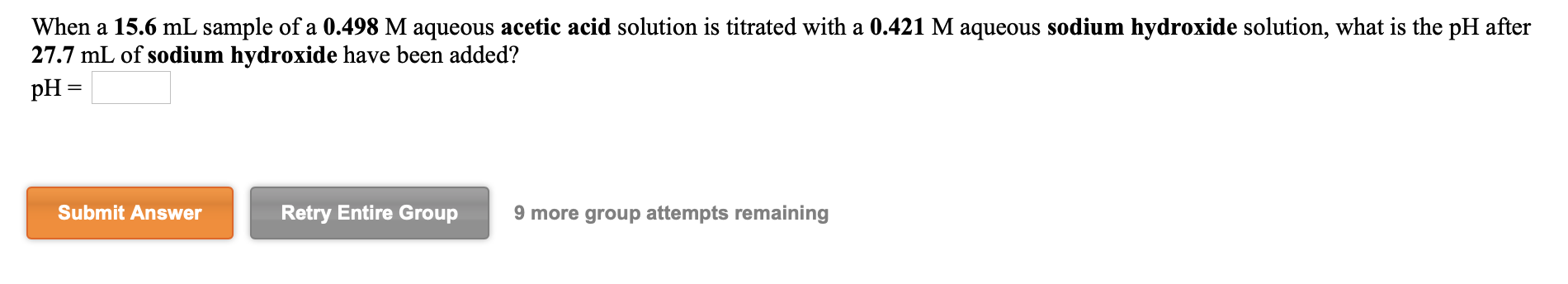When a 15.6 mL sample of a 0.498 M aqueous acetic acid solution is titrated with a 0.421 M aqueous sodium hydroxide solution, what is the pH after 27.7 mL of sodium hydroxide have been added? pH = Submit Answer Retry Entire Group 9 more group attempts remaining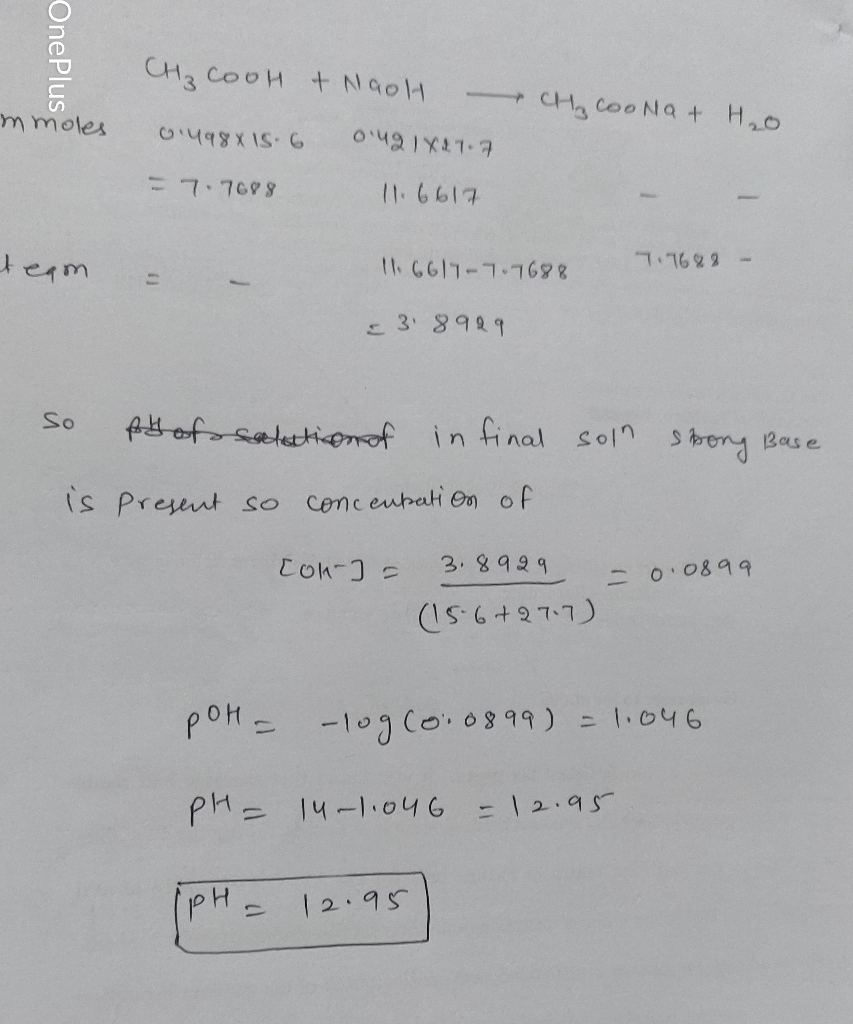#### Earn Coins

Coins can be redeemed for fabulous gifts.

Similar Homework Help Questions
• ### When a 17.5 mL sample of a 0.433 M aqueous acetic acid solution is titrated with...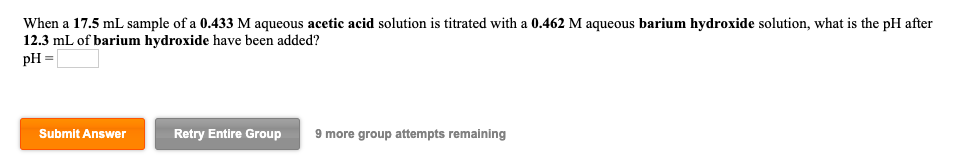When a 17.5 mL sample of a 0.433 M aqueous acetic acid solution is titrated with a 0.462 M aqueous barium hydroxide solution, what is the pH after 12.3 mL of barium hydroxide have been added? pH = Submit Answer Retry Entire Group 9 more group attempts remaining A 36.7 mL sample of a 0.371 M aqueous acetic acid solution is titrated with a 0.377 M aqueous sodium hydroxide solution. What is the pH after 10.9 mL of base have...

• ### A 23.0 mL sample of a 0.497 M aqueous acetic acid solution is titrated with a...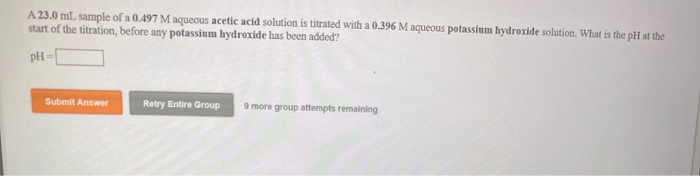A 23.0 mL sample of a 0.497 M aqueous acetic acid solution is titrated with a 0.396 M aqueous potassium hydroxide solution. What is the pH at the start of the titration, before any potassium hydroxide has been added? PH Submit Answer Retry Entire Group 9 more group attempts remaining What is the pH at the equivalence point in the titration of a 25.5 mL sample of a 0.411 M aqueous acetic acid solution with a 0.352 M aqueous barium...

• ### When a 17.3 mL sample of a 0.447 M aqueous hydrocyanic acid solution is titrated with...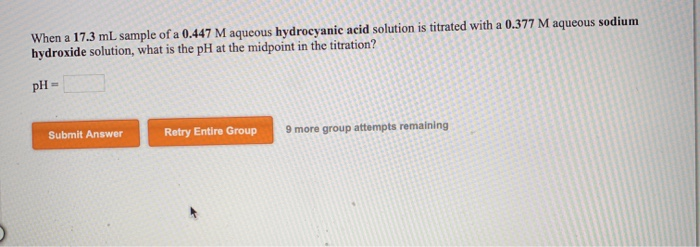When a 17.3 mL sample of a 0.447 M aqueous hydrocyanic acid solution is titrated with a 0.377 M aqueous sodium hydroxide solution, what is the pH at the midpoint in the titration? pH = Submit Answer Retry Entire Group 9 more group attempts remaining A 36.4 mL sample of a 0.391 M aqueous hydrofluoric acid solution is titrated with a 0.250 M aqueous sodium hydroxide solution. What is the pH after 17.9 mL of base have been added? pH...

• ### When a 23.0 mL sample of a 0.311 M aqueous nitrous acid solution is titrated with...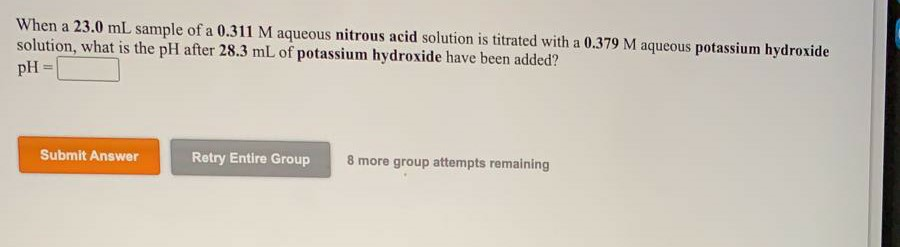When a 23.0 mL sample of a 0.311 M aqueous nitrous acid solution is titrated with a 0.379 M aqueous potassium hydroxide solution, what is the pH after 28.3 mL of potassium hydroxide have been added? pH = Submit Answer Retry Entire Group 8 more group attempts remaining

• ### this question. When a 21.0 mL sample of a 0.411 M aqueous hydrocyanic acid solution is...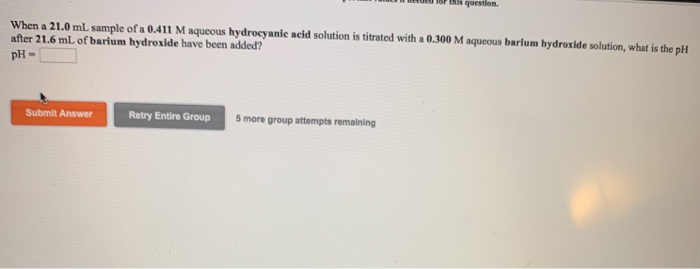this question. When a 21.0 mL sample of a 0.411 M aqueous hydrocyanic acid solution is titrated with a 0.300 M aqueous barium hydroxide solution, what is the pH after 21.6 mL of barium hydroxide have been added? PH Submit Answer Retry Entire Group 5 more group attempts remaining What is the pH at the equivalence point in the titration of a 23.3 ml sample of a 0.347 M aqueous hypochlorous acid solution with a 0.395 M aqueous barium hydroxide...

• ### A 35.7 mL sample of a 0.480 M aqueous hypochlorous acid solution is titrated with a...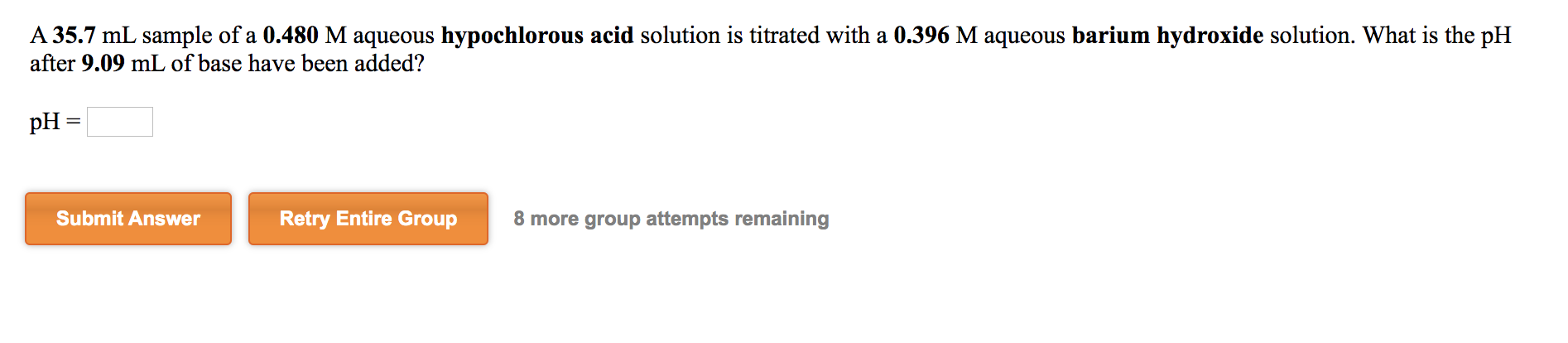A 35.7 mL sample of a 0.480 M aqueous hypochlorous acid solution is titrated with a 0.396 M aqueous barium hydroxide solution. What is the pH after 9.09 mL of base have been added? pH = Submit Answer Retry Entire Group 8 more group attempts remaining

• ### help A 33.3 mL sample of a 0.482 M aqueous hydrocyanic acid solution is titrated with...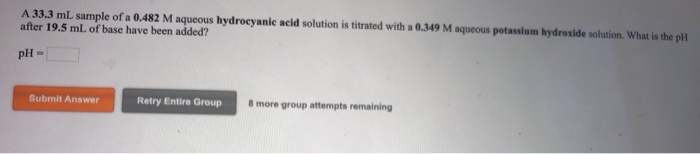help A 33.3 mL sample of a 0.482 M aqueous hydrocyanic acid solution is titrated with a 0.349 M aqueous potassium hydroxide solution. What is the pil after 19.5 mL of base have been added? pH- Submit Answer Retry Entire Group 8 more group attempts remaining

• ### When a 28.9 mL sample of a 0.433 M aqueous hydrofluoric acid solution is titrated with...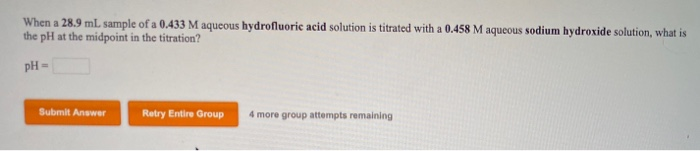When a 28.9 mL sample of a 0.433 M aqueous hydrofluoric acid solution is titrated with a 0.458 M aqueous sodium hydroxide solution, what is the pH at the midpoint in the titration? pH- Submit Answer Bubmit rower Retry Entire Group Retry Entero Group 4 more group attempts remaining

• ### A45.2 ml sample of a 0.329 M aqueous hydrocyanic acid solution is titrated with a 0.203...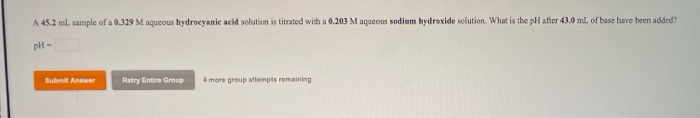A45.2 ml sample of a 0.329 M aqueous hydrocyanic acid solution is titrated with a 0.203 M aqueous sodium hydroxide solution. What is the pH after 43.0 mL of base have been added? pH- B Answer Ratry Entire Group 4 more group attempts remaining

• ### When a 19.0 mL sample of a 0.462 M aqueous hypochlorous acid solution is titrated with a 0.371 M aqueous barium hyd...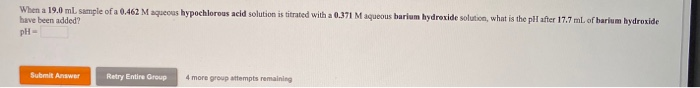When a 19.0 mL sample of a 0.462 M aqueous hypochlorous acid solution is titrated with a 0.371 M aqueous barium hydroxide solution, what is the pH after 17.7 ml. of harium hydroxide have been added pH- SA Retry Entire Group more greatempts remaining